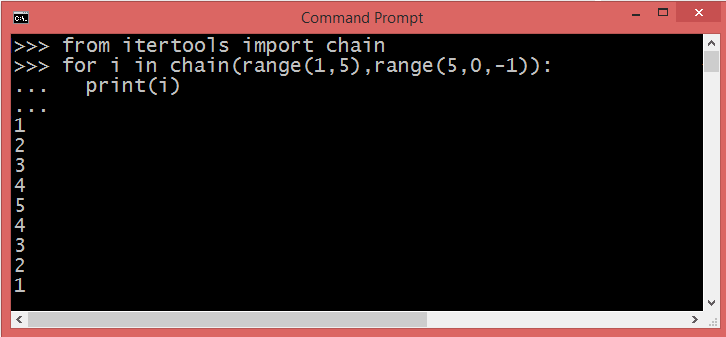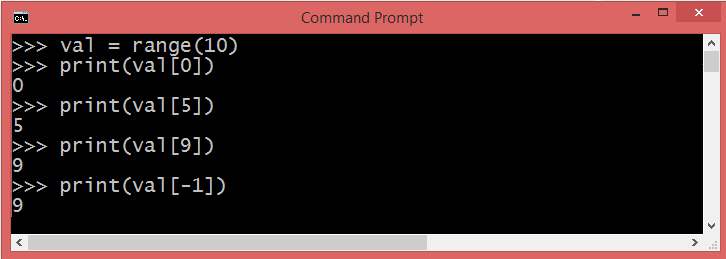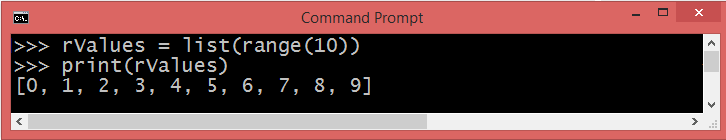Net-informations.com

# Python range() function

The Python range() function (built-in-function) is used to generate a sequence of numbers. It generates a list of integers from some lower limit (0 by default) up to some upper limit , possibly in increments (steps) of some other number (1 by default). The range() function works a little bit differently between Python 2.x and Python 3.x under the hood, however the concept is the same.

#### Syntax

1. start - The starting point for the range() to generate numbers.
2. stop - It is the point just before range() ends. The range of integers end at stop - 1.
3. step (Optional) - integer value which determines the increment between each integer in the sequence.

## How to floats with Python's range() function

Unfortunately Python range() function doesn't support the float numbers. i.e. user cannot use floating point number in any of its argument.If you provide a non-integer stop value, then it raises a TypeError: 'float' object cannot be interpreted as an integer .

However, you can implement an alternate way to get the range of floating numbers .

## Python while loop using range() function## Concatenation of two range() functions using chain() method

The chain() method takes any number of iterables as arguments and "chains" them together.## Accessing Python range() values using index## Python range() to List

The Python range type represents an immutable sequence of numbers, so it is possible to convert the output of a range() to the Python list.net-informations.com (C) 2022    Founded by raps mk